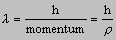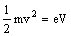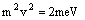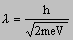9.2.6 Wave particle duality  link to a cheesy web site

Many of light’s properties can be explained by considering it to be a transverse wave motion. Reflection, refraction, diffraction, interference and polarisation can all be satisfactorily explained. However the photoelectric effect poses problems because it is necessary to attribute particle like properties to light in order to explain this phenomena.

Note:  remember that the wave model and the particle model are exactly that – just models which help us to explain the behaviour of light. Neither of them is a literal description of what light is.

#### De Broglie’s theory

In 1924, in his PhD thesis Louis de Broglie wrote,

‘... I assumed that the existence of waves and particles, perceived by Einstein in 1905 in respect of light in his theory of light quanta, should be extended to all types of particle in the form of coexistence of a physical wave with a particle incorporated in it....’

De Broglie was suggesting that all matter should have both a wave and a particle characteristic. Other than light there was no experimental work to justify this- it was pure speculation. He suggested that the wavelength of all matter was given by the relation(this is arrived at by equating E=hf and E=mc2)

where     r = mv, the particle’s momentum

This equation was confirmed by experiments carried out on electron diffraction by Davisson and Germer in 1927.

#### Electron diffraction experiments

When an electron is accelerated through a pd V the work done on the electron is eV. If the energy gained by the electron is entirely kinetic, thenand thusfrom which it can be shown thatIt can thus be shown that the wavelength of an electron accelerated through a pd of 100V will be approximately 1.2 x 10-10m. This is approximately the same value as the wavelength of X-rays, which can be diffracted by metal crystals. Thus metal crystals ought to be able to diffract electrons too.

In 1927 Davisson and Germer showed that a beam of electrons directed at a metal crystal produced a diffraction pattern similar to an X ray diffraction pattern. In the same year George Thomson (son of J.J) showed that a beam of electrons directed at a thin polycrystalline metal foil produced a diffraction pattern of rings. An X ray beam directed at the foil produced the same pattern.

Thus de Broglie was proved correct. Particles do have a wave-like nature.

#### Double slit interference using electrons and the effect of using high and low intensity beams

Using a similar set up to Young’s slits, but using electrons instead of light it is possible to obtain an interference pattern similar to that produced by light except that the fringes are about 1000 times closer together.

The easiest interpretation is that half the electrons arrive at each slit and interference occurs between these two sets of electrons. This is a false interpretation.

It is found that if the intensity of the electron beam is reduced until only one electron at a time is released, the interference pattern still occurs. It can be concluded from this result that each electron interferes with itself and therefore each electron actually passes through both slits. By considering the electron as a particle this explanation appears highly implausible but if one considers it’s wave-like nature then the explanation does become more plausible.

Comparison with light photons

Light is made up of photons each of which travels in a specific direction. Since interference requires coherent sources, and since the arrival of individual photons or electrons at a slit is random, for coherence to occur then each photon or electron must interfere with itself. Thus when considering the interference of light one must accept that each photon passes through each slit and interferes with itself.

#### Probabilistic interpretation of interference

After passing through the double slits it is impossible to predict which direction each individual electron or photon will travel in. However it is possible to predict the probability of an electron arriving at a specific point on the screen. Thus the central point of a bright fringe indicates a high probability of finding an electron (or photon) and the central part of a dark fringe indicates a low probability.

next